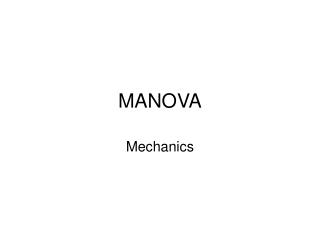Download PresentationMANOVA

# MANOVA - PowerPoint PPT PresentationDownload Presentation## MANOVA

- - - - - - - - - - - - - - - - - - - - - - - - - - - E N D - - - - - - - - - - - - - - - - - - - - - - - - - - -
##### Presentation Transcript

1. MANOVA Mechanics

2. MANOVA is a multivariate generalization of ANOVA, so there are analogous parts to the simpler ANOVA equations • First lets revisit Anova • Anova tests the null hypothesis • H0: 1= 2… = k • How do we determine whether to reject?

3. SSTotal = • SSbg = • SSwg =

4. Steps to MANOVA • When you have more than one IV the interaction looks something like this: • SSbg breaks down into main effects and interaction

5. With one-way anova our F statistic is derived from the following formula

6. Steps to MANOVA • The multivariate test considers not just SSb and SSw for the dependent variables, but also the relationship between the variables • Our null hypothesis also becomes more complex. Now it is

7. With Manova we now are dealing with matrices of response values • Each subject now has multiple scores, there is a matrix, as opposed to a vector, of responses in each cell • Matrices of difference scores are calculated and the matrix squared • When the squared differences are summed you get a sum-of-squares-and-cross-products-matrix (S) which is the matrix counterpart to the sums of squares. • The determinants of the various S matrices are found and ratios between them are used to test hypotheses about the effects of the IVs on linear combination(s) of the DVs • In MANCOVA the S matrices are adjusted for by one or more covariates

8. We’ll start with this matrix Now consider the matrix product, X'X. • The result (product) is a square matrix. • The diagonal values are sums of squares and the off-diagonal values are sums of cross products. The matrix is an SSCP (Sums of Squares and Cross Products) matrix. • So Anytime you see the matrix notation X'X or D'D or Z'Z, the resulting product will be a SSCP matrix.

9. Manova • Now our sums of squares goes something like this • T = B + W • Total SSCP Matrix = Between SSCP + Within SSCP • Wilk’s lambda equals |W|/|T| such that smaller values are better (less effect attributable to error)

10. We’ll use the following dataset • We’ll start by calculating the W matrix for each group, then add them together • The mean for Y1 group 1 = 3, Y2 group 2 = 4 • W = W1 + W2 + W3 Group Y1 Y2 1 2 3 1 3 4 1 5 4 1 2 5 2 4 8 2 5 6 2 6 7 3 7 6 3 8 7 3 9 5 3 7 6 Means 5.67 5.75

11. So • We do the same for the other groups • Adding all 3 gives us

12. Now the between groups part • The diagonals of the B matrix are the sums of squares from the univariate approach for each variable and calculated as:

13. The off diagonals involve those same mean differences but are the products of the differences found for each DV

14. Again T = B + W • And now we can compute a chi-square statistic* to test for significance

15. Same as we did with canonical correlation (now we have p = number of DVs and k = number of groups)

16. Test Statistic – Wilk’s Lambda • Approximate Multivariate F for Wilk’s Lambda is

17. Effect size • Already have it • Eta-squared • Partial Eta-squared • *Eta-squared is actually equal to the squared multiple (canonical) correlation between the dummy coded categorical variable and DVs for the first function • Again, it’s canonical correlation with (coded) categorical variables in one set